V.C. Source Detection

# IRAS Explanatory Supplement V. Data Reduction C. Source Detection

Chapter Contents | Introduction | Authors | References

The calibrated raw data for each of the 59 operating detectors were examined for point sources and small extended sources. The detection of the latter is described in Section V.E.1. For each observation the accepted point source detections were passed, with detector number, time of detection (and uncertainty), flux (and uncertainty), signal-to-noise ratio (SNR), and the correlation coefficient with the point source template (CC, see below), to the seconds-confirmation processor (Section V.D.2). A noise history was also created for each detector. If a detection occurred in a one-second period in which the analog-to-digital converter was saturated, then the detection was flagged.

## C.1 Square Wave Filter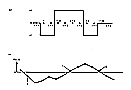Figure V.C.1 a) An eight-point zero-sum, square-wave filter was applied to the data streams (top panel); b) The detection processor looked for positive square wave peaks between zero crossings in the filtered data stream (bottom panel). larger largest

E(x,i) = -Xi - Xi+1 + Xi+2 + Xi+3 + Xi+4 + Xi+5 - Xi+6 - Xi+7

(V.C.1)

This square-wave filter was applied at each point in the data stream, and a search was made for positive square-wave excursions between zero crossings, defined as a pair of data points (i,j) such that (see Fig. V.C.1b):
 E(x,i) > 0; E(x,k) >/- 0 for i
That is, find the values n, i, j such that: n with E(x,n) < 0; the first i > n with E(x,i) > 0; and the first j > i with E(x,j+1) < 0. The positive excursion (i,j) has a peak at the first p with i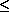pj, such that E(x,p) is maximal among E(x,i),..., E(x,j). Peaks with square-wave amplitudes, E(n,p), greater than 2.5 times the noise Nx were passed on as candidates for point sources.

## C.2 Noise Estimator

The noise Nx for a data stream was defined as the median of all E(x,p) for square-wave peaks p. Such positive square-wave excursions occurred about once every 6 samples. It was found from prelaunch simulations and from analysis of in-flight data that this median noise estimator gave a reasonable representation of the rms noise, in the sense thatrms1.2 Nx

(V.C.2)

The enormous volume of data meant that determination of a running estimate of the rms noise would have involved a prohibitive computational run time.

The initial value of Nx was the median of the first 50 square-wave peaks. Nx was then updated at every square-wave peak E(x,p) as follows:

If E(x,p) < Nx then reduce Nx by the factor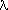(< 1); otherwise, increase Nx by the factor 1/.

The parametercontrolled the stability of the noise estimator. Asapproached 1, the noise estimator became very stable, but it also lagged behind any change in the noise by about 5/(1 -) samples. In tuning the value ofgreat importance was attached to achieving a stable noise estimate at high Galactic latitudes, where the noise was mainly due to detector noise, and a value= 0.95 was set at 12, 25 and 60 µm, and 0.90 at 100 µm. This meant that the noise estimate lagged by about 25', 25', 50', 50' at 12, 25, 60 and 100 µm. Regions with steep gradients in the density of point sources, such as the Galactic plane, had large gradients in the noise amplitude. Hence the noise was underestimated as the plane was approached and overestimated after it was passed (Sections V.C.7, VIII.D.6). This error was very large, and since sources were thresholded partly on signal-to-noise ratio (Section V.C.4, below), the effective threshold was raised to very large values after passing the Galactic plane, resulting in a shadow zone in which few sources were accepted. To keep the extent of the 100 µm shadow zone no larger than that at 60 µm,was set to 0.90 at 100 µm compared with 0.95 at 60 µm. However, this adversely affected the stability of the 100 µm noise estimate in the presence of cirrus at higher Galactic latitudes, resulting in the rejection of some detections that should have been accepted and hence a reduction in completeness of the catalog at 100 µm (Section VIII.D).

The noise estimate was maintained in a noise history file for each detector after multiplication by the factor to convert it to an estimate of the rms noise on a single sample. To compress the size of this file, an entry was made only if linear extrapolation of the previous two entries would lead to an error greater than 35%.

## C.3 Timing Estimate

The time of the square-wave peak at E(x,p) was estimated from the maximum of the parabola passing through the three points (p - 1,E(x,p - 1)), (p,E(x,p)), (p + 1, E(x,p + 1)). The delay between a source in the unfiltered data and its peak in the square-wave function was subtracted from the estimate to give the detection time. A small offset to account for electronic delay and the sampling time of the detector was included. The timing uncertainty was taken from a look-up table as a function of the values of signal-to-noise ratio and correlation coefficient for the source.

## C.4 Correlation with Point Source Template

The heart of the point source detection processor was the comparison of the data for candidate sources selected by the square-wave filter with the profile, or template, expected for an ideal point source. For this purpose the 11 samples centered on the candidate detection time, Yi, i = 0...10, were compared with the appropriately shifted template Ri superimposed on a linear baseline. The amplitude A of the detection was determined from fitting the 11 data values Yi to the function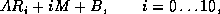(V.C.3)

where B is the baseline height and M is its slope, A, M, and B were determined by the method of least squares, i.e., by minimizing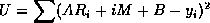(V.C.3.2)

where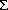stands for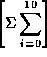(V.C.3.3)

Thus,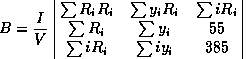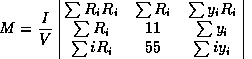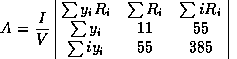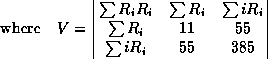(V.C.4.1)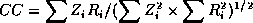(V.C.5)

(V.C.5.1)

(i)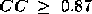(V.C.6.1)

and

(ii)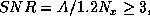,

(V.C.6.2)

The total rms uncertainty in amplitude, A, over the 11 data samples can be shown to be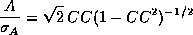(V.C.7)

Thus the correlation coefficient is a measure of the local signal-to-noise ratio and a threshold of 0.87 corresponds to a signal-to-noise ratio of about 5.7. In regions where the noise was roughly independent of time, the main thresholding was therefore provided by the correlation coefficient. The square-wave filter threshold (Section V.C.1 above) was set low so that as few acceptable detections as possible were rejected, within the constraints of the available computer time. It should be noted that a low correlation coefficient for a bright point-source is probably an indication that the source is slightly extended. In regions of high source density (see Section V.H.6), where extended structure is a considerable problem, the correlation coefficient threshold was increased to 0.97.

## C.5 Determination of Templates

Immediately after launch, predicted detector responses to an ideal point source were used. Composite templates were constructed for each detector using sources detected with high correlation coefficient and signal-to-noise ratio. The a priori templates were replaced with the composite templates and the analysis repeated, using 12 hours worth of data. Convergence was achieved after only a few iterations. Figure V.C.2 shows representative point source templates for one detector in each wavelength band. Since no evidence for detector-to-detector variation within a band was found, the results for all the detectors in each band were averaged together to produce the final composite templates.

## C.6 Low Signal-to-Noise Detections

A secondary class of detections called low signal-to-noise detections was defined as those with signal-to-noise ratios between 3 and the threshold required for a valid detection. Because the threshold for valid detections was itself set at 3, no low signal-to-noise detections should have been generated. However, due to the round-off errors in the computation of the signal-to-noise ratio, a few were created. These were only used to provide upper limits for sources confirmed in other bands.